• Assertions
• MergeSort
• Botton-up MergeSort
• Sorting Complexity
• Java: Comparator interface
• Stability

# Assertions

``````// after sort
assert isSorted(a, lo, hi);
``````

Can enable or disable at runtime

``````# enable assertions
\$ java -ea MyProgram

# disable assertions (default)
\$ java -da MyProgram
``````

## Best practices

• Use assertions to check internal invariants
• assume assertions will be disabled in production code
• do not use for external argument checking

# MergeSort

Animation: Merge Sort

## Implement

``````public class MergeSort{
/**
* Interface
**/
public static void mergeSort(Comparable[] a){
Comparable[] aux = new Comparable[a.length];
mergeSort(a, aux, 0, a.length -1);
}
/**
* Recursion method
*
* Parameters:
* a: data
* aux: buffer
* lo: sort start at
* hi: sort end with
**/
private static void mergeSort(Comparable[] a, Comparable[] aux, int lo, int hi){
if (lo >= hi) return;
int mid = (lo + hi) / 2;
p("mergeSort: [" + lo + ", " + mid + "]");
mergeSort(a, aux, lo, mid);
p("mergeSort: [" + (mid+1) + ", " + hi + "]");
mergeSort(a, aux, mid + 1, hi);
p("merge: [" + lo + ", " + mid + "] + [" + (mid+1) + ", " + hi + "] -> [" + lo + ", " + hi + "]");
merge(a, aux, lo, mid, hi);
}
/**
* Merge two sub-arrays into one: aux[lo, mid] + aux[mid + 1, hi] -> arr[lo, hi]
*
* Parameters:
* a: target data
* aux: source data, buffer
* lo: source 1 start at
* mid: source 1 end with, source 2 start at mid+1
* hi: source 2 end with
**/
private static void merge(Comparable[] a, Comparable[] aux, int lo, int mid, int hi){
for (int i = lo; i <= hi; i++){
aux[i] = a[i];
}
// count: point to target array
int count = lo;
// lm: point to arr[lo, mid]
int lm = lo;
// mh: point to arr[mid+1, hi]
int mh = mid + 1;

while(count <= hi){
if (lm > mid)                       a[count++] = aux[mh++];
else if (mh > hi)                   a[count++] = aux[lm++];
else if (less(aux[lm], aux[mh]))    a[count++] = aux[lm++];
else                                a[count++] = aux[mh++];
// == else if (aux[lo] > aux[mid + 1])
}
}
public static boolean less(Comparable a, Comparable b){
return a.compareTo(b) < 0;
}
// unit test
public static void main(String[] args){
// int[] will cause error, since int is not Comparable. Auto-boxing do not cover this case
Integer[] a = {4,2,52,3,5,1,2,23,9,20,0};
mergeSort(a);
for (int i : a){
System.out.print(i + ", ");
}
System.out.println();
}
public static void p(String s){
System.out.println(s);
}
}
``````

## Cost

Time

Mergesort uses at most N lg N compares and 6 N lg N array accesses to sort any array of size N

Space

Mergesort uses extra space proportional to N.

A sorting algorithm is in-place if it uses ≤ c log N extra memory. Ex. Insertion sort, selection sort, shellsort.

## Improvements

Use insertion sort for small subarrays.

• Mergesort has too much overhead for tiny subarrays.
• Cutoff to insertion sort for ≈ 7 items.
``````private static void cutOffSort(Comparable[] a, Comparable[] aux, int lo, int hi) {
int CUTOFF = 7; // 7 is fine
// length = hi - lo + 1
if (hi - lo + 1 <= CUTOFF) {
Insertion.sort(a, lo, hi);
return;
}
int mid = lo + (hi - lo) / 2;
sort (a, aux, lo, mid);
sort (a, aux, mid+1, hi);
merge(a, aux, lo, mid, hi);
}
``````

will make it maybe 20% faster.

Stop if sorted: max item of first half is smaller than right half.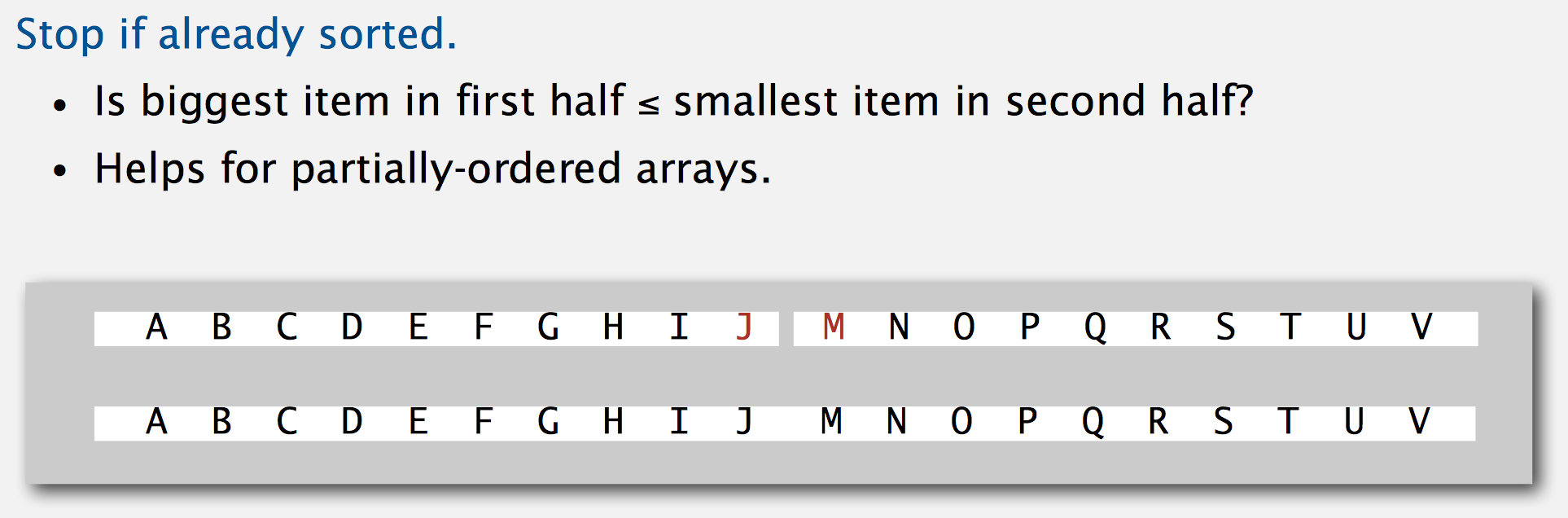``````private static void mergeSort(Comparable[] a, Comparable[] aux, int lo, int hi){
if (lo >= hi) return;
int mid = (lo + hi) / 2;
mergeSort(a, aux, lo, mid);
mergeSort(a, aux, mid + 1, hi);
// max item of first half is smaller than right half, so it is already sorted
if (less(a[mid], a[mid + 1])) return;
merge(a, aux, lo, mid, hi);
}
``````

Eliminate the copy to the auxiliary array.

// TODO: 不懂？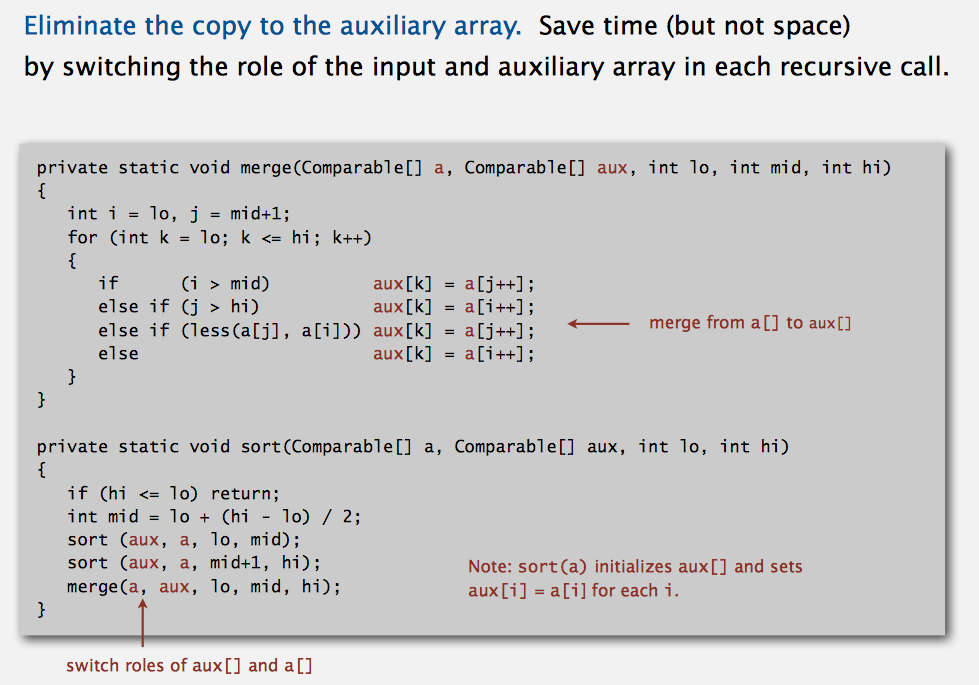# Botton-up MergeSort

Remove recursive.

``````public static void mergeSortWithoutRecursive(Comparable[] a){
int N = a.length;
Comparable[] aux = new Comparable[N];
for (int sz = 1; sz < N; sz *= 2){
for (int lo = 0; lo < N - sz; lo += sz*2)
merge(a, aux, lo, lo+sz-1, Math.min(lo+2*sz-1, N-1));
}// end for size
}
``````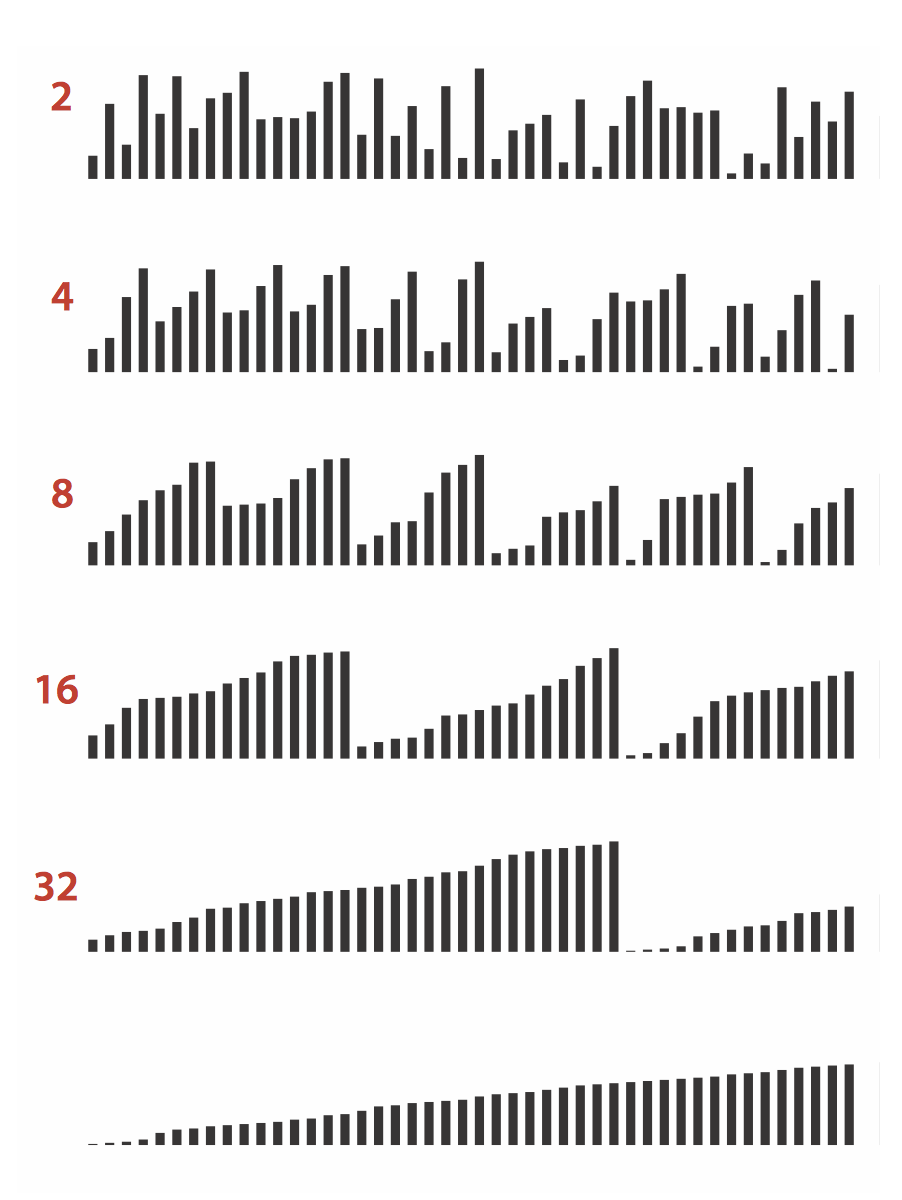• It does not always divide by half. So a part might be small than other(s).
• 10% slower than recursive, top-down mergesort on typical systems
• get log(2)(n) passes(遍历)

# Complexity

• Model of computation: Allowable operations

• Cost model: Operation count(s)

• Upper bound: Cost guarantee provided by some algorithm for X (current algorithms can achieve)

• Lower bound: Proven limit on cost guarantee of all algorithms for X (future possible algorithms can maximumly achieve)

• Optimal algorithm: Algorithm with best possible cost guarantee for X (this algorithm is the best)

## Sort Complexity

• Model of computation: decision tree (can access information only through compares; example below)
• Cost model: # compares.
• Upper bound: ~ N lg N from mergesort
• Lower bound: N lg N
• Optimal algorithm: mergesort

### Decision tree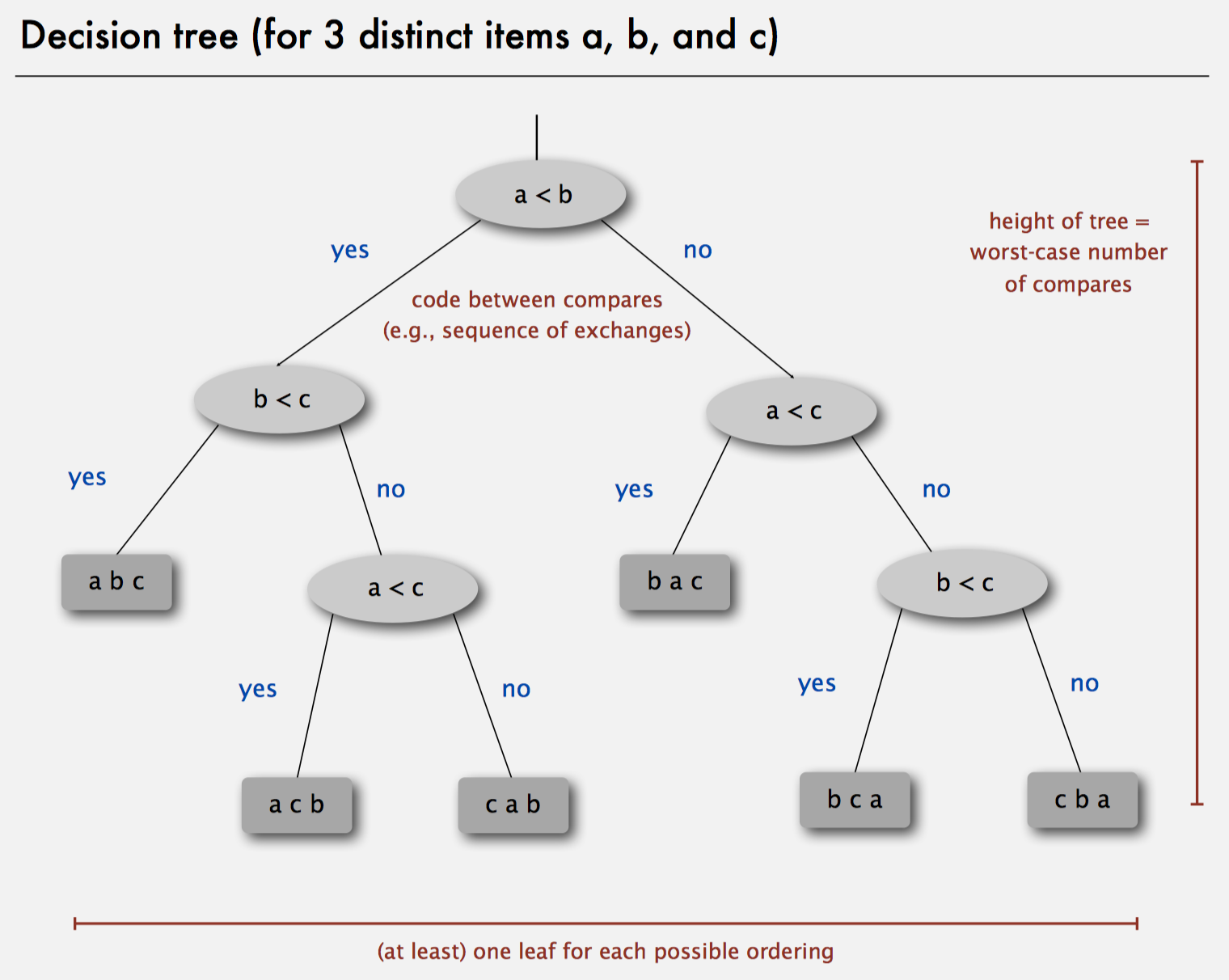• Height: Worst case: 3 compares. Aka, the height of decision tree.
• Leaf: each possible ordering

## Lower bound

We can proof that any compare-based sort algorithm must use at least N lg N compares in the worst-case.

Pf.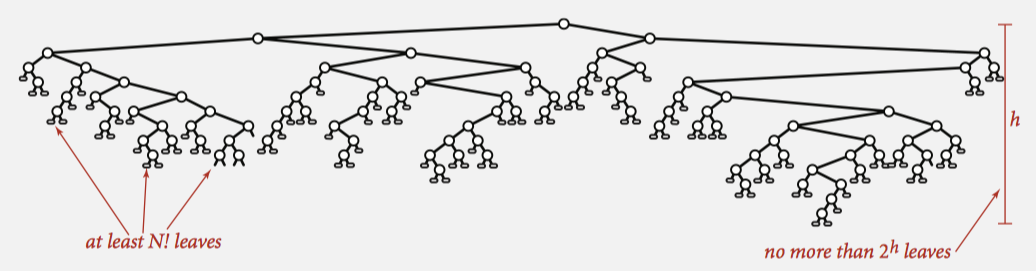leaves = N! max hight = lg N! (log(2)(N!)) => lg(N!) = N lg N (Stirling’s formula)

## Optimal algorithm

Since mergesort has a cost of N lg N in the worst-case. So it’s the best algorithm. But mergesort is not good at Space cost. It cost twice N.

In some particular cases, MergeSort may not be the best:

• Partially-ordered arrays. Depending on the initial order of the input, we may not need N lg N compares. Ex. insertion sort requires only N-1 compares if input array is sorted.

# Java: Comparator interface

Usage:

``````public static void sort(Object[] a, Comparator comparator) {
int N = a.length;
for (int i = 0; i < N; i++)
for (int j = i; j > 0 && less(comparator, a[j], a[j-1]); j--)
exchange(a, j, j-1);
}

private static boolean less(Comparator c, Object v, Object w) { return c.compare(v, w) < 0; }

private static void exchange(Object[] a, int i, int j) { Object swap = a[i]; a[i] = a[j]; a[j] = swap;
``````

Implement:

``````public class Student {

public static final Comparator<Student> BY_NAME = new ByName();
public static final Comparator<Student> BY_SECTION = new BySection();
private final String name;
private final int section;

private static class ByName implements Comparator<Student> {
public int compare(Student v, Student w) {
return v.name.compareTo(w.name); }
}
…
private static class BySection implements Comparator<Student> {
public int compare(Student v, Student w) {
return v.section - w.section; }
}
``````

# Stability

Ex. for unstable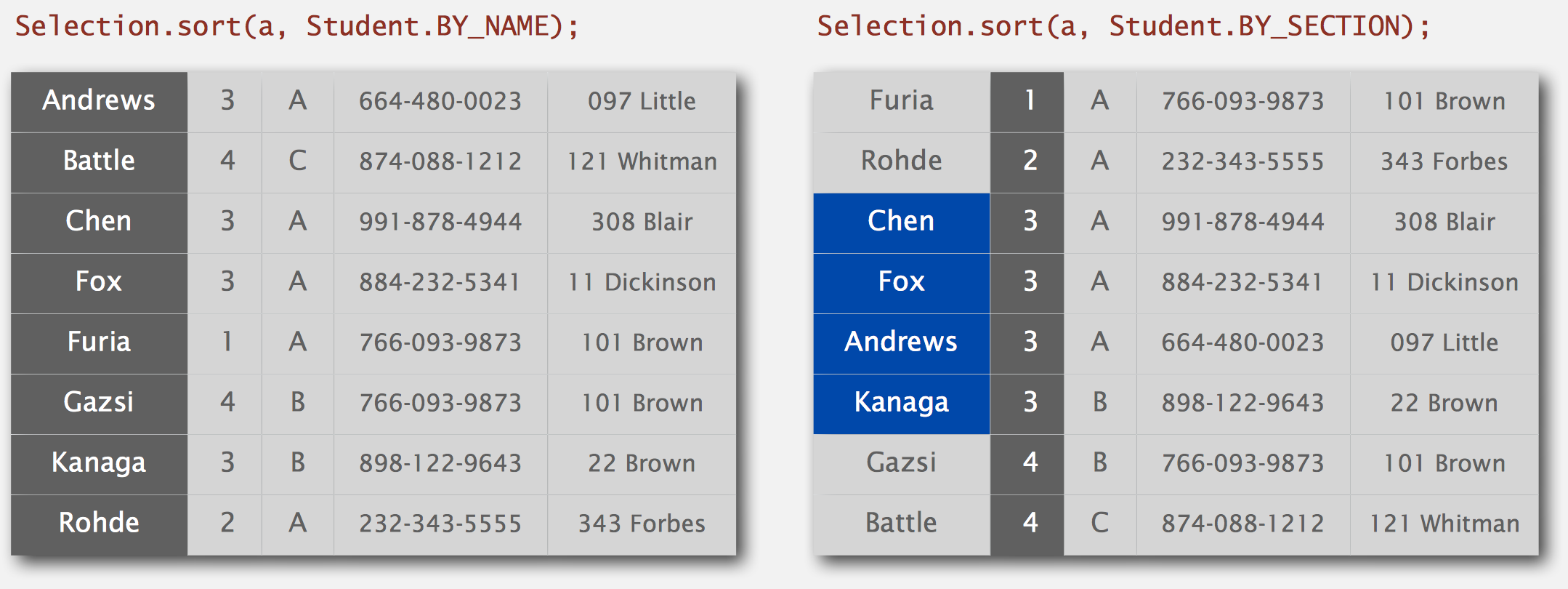After sort, students in `section 3` no longer stay sorted by name.

## What is Stable?

• A stable sort preserves the relative order of items with equal keys
• Never move equal items pass one another

Stable sort:

• insertion sort
• mergesort

Unstable sort:

• selection sort
• shellsort

## Why is Selection Sort not stable?

A small example:

``````Let b = B in

< B > , < b > , < a > , < c > (with a < b < c)

After one cycle the sequence is sorted but the order of B and b has changed:

< a > , < b > , < B > , < c >
``````